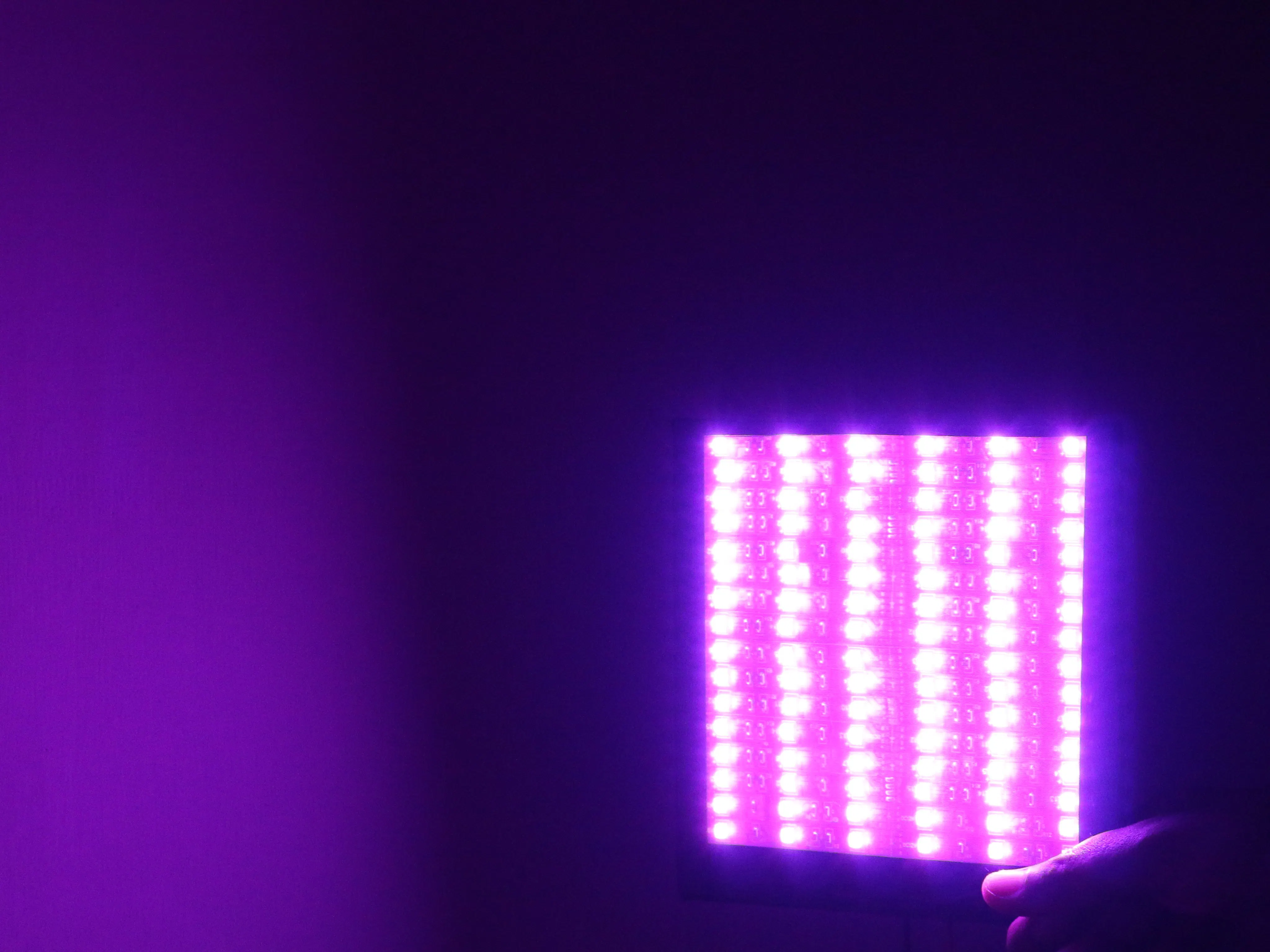Project tutorialRGB Camera Backlight Control With Android Mobile

RGB LED connected with Arduino Nano and Bluetooth module and control with Android mobile app.

• 1,949 views
• 1 comment
• 8 respects

Necessary tools and machinesSoldering iron (generic)

Apps and online services

RGB lights Compaing three LED lights Red,Green & Blue. We adjust the brightness of the LED and it creates new colours. So the LED adjusting brightness is done using codes(0-255).

• As the LEDs are very close to each other, we can only see the final colors result rather than the three colors individually.
• To have an idea on how to combine the colors, take a look at the following chart. This is the simplest color mixing chart, there are more complex color charts on the web.
• RGB LEDs have 4 pins which can be distinguished by their length. The longest one is the ground (-) or voltage (+) depending if it is a common cathode or common anode LED, respectively.

RGB LED is a combination of 3 LEDs in just one package:

• 1x Red LED
• 1x Green LED
• 1x Blue LED

The color produced bythe RGB LED is a combination of the colors of each one of these three LEDs.

Mixing colors

To produce other colors, you can combine the three colors in different intensities. To generate different colors you can use PWM to adjust the brightness of each LED. As the LEDs are very close to each other, we can only see the final colors result rather than the three colors individually.

R     G     B
(255,255,255)= White color

The 255 is full brightness of the led light

RGB LED Two Types:

int redPin = 11;
int greenPin = 10;
int bluePin = 9;
void setup()
{
pinMode(redPin, OUTPUT);
pinMode(greenPin, OUTPUT);
pinMode(bluePin, OUTPUT);
}
void loop()
{
setColor(255, 0, 0);  // red
delay(1000);
setColor(0, 255, 0);  // green
delay(1000);
setColor(0, 0, 255);  // blue
delay(1000);
setColor(255, 255, 0);  // yellow
delay(1000);
setColor(80, 0, 80);  // purple
delay(1000);
setColor(0, 255, 255);  // aqua
delay(1000);
}
void setColor(int red, int green, int blue)
{
#ifdef COMMON_ANODE
red = 255 - red;
green = 255 - green;
blue = 255 - blue;
#endif
analogWrite(redPin, red);
analogWrite(greenPin, green);
analogWrite(bluePin, blue);
}

Arduino Uno using Bluetooth RGB Controller:

int color =0;
int red = 12;
int green =11;
int blue =10;
void setup() {
Serial.begin(9600);
pinMode(red, OUTPUT);
pinMode(green, OUTPUT);
pinMode(blue, OUTPUT);
analogWrite(red,0);
analogWrite(green,0);
analogWrite(blue,0);
}
void loop(){
if(Serial.available()>0){
char Rec = char(color);
if (Rec != '0')
{
Serial.println(Rec);
}
}
//Black
if (color == 'B')
{
analogWrite(red,0);
analogWrite(green,0);
analogWrite(blue,0);
}
//WHITE
if (color == 'W')
{
analogWrite(red,255);
analogWrite(green,255);
analogWrite(blue,255);
}
//RED
if (color == 'R')
{
analogWrite(red,255);
analogWrite(green,0);
analogWrite(blue,0);
}
//LIME
if (color == 'L')
{
analogWrite(red,0);
analogWrite(green,255);
analogWrite(blue,0);
}
//BlUE
if (color == 'E')
{
analogWrite(red,0);
analogWrite(green,0);
analogWrite(blue,255);
}
//Yellow
if (color == 'Y')
{
analogWrite(red,255);
analogWrite(green,255);
analogWrite(blue,0);
}
//Cyan/Aqua
if (color == 'C')
{
analogWrite(red,0);
analogWrite(green,255);
analogWrite(blue,255);
}
//Magenta / Fuchsia
if (color == 'M')
{
analogWrite(red,255);
analogWrite(green,0);
analogWrite(blue,255);
}
// Maroon
if (color == 'F')
{
analogWrite(red,128);
analogWrite(green,0);
analogWrite(blue,0);
}
// Olive
if (color == 'O')
{
analogWrite(red,128);
analogWrite(green,128);
analogWrite(blue,0);
}
//Green
if (color == 'G')
{
analogWrite(red,0);
analogWrite(green,128);
analogWrite(blue,0);
}
// Purple
if (color == 'P')
{
analogWrite(red,128);
analogWrite(green,0);
analogWrite(blue,128);
}
//  Navy
if (color == 'N')
{
analogWrite(red,0);
analogWrite(green,0);
analogWrite(blue,128);
}
//light coral
if (color == 'J')
{
analogWrite(red,240);
analogWrite(green,128);
analogWrite(blue,128);
}
//orange red
if (color == 'X')
{
analogWrite(red,255);
analogWrite(green,69);
analogWrite(blue,0);
}
//green yellow
if (color == 'G')
{
analogWrite(red,173);
analogWrite(green,255);
analogWrite(blue,47);
}
// spring green
if (color == 'S')
{
analogWrite(red,0);
analogWrite(green,255);
analogWrite(blue,127);
}
//   aqua marine
if (color == 'A')
{
analogWrite(red,127);
analogWrite(green,255);
analogWrite(blue,212);
}
//   hot pink
if (color == 'H')
{
analogWrite(red,255);
analogWrite(green,105);
analogWrite(blue,180);
}
// honeydew
if (color == 'D')
{
analogWrite(red,240);
analogWrite(green,255);
analogWrite(blue,240);
}
// light gray / light grey
if (color == 'U')
{
analogWrite(red,211);
analogWrite(green,211);
analogWrite(blue,211);
}
}

Code

Android Motion Detector Camera with Arduino/MCU

Project tutorial by Walid Mafuj

• 5,986 views
• 22 respects

The Magnetic Field and RGB Tester

Project tutorial by Kutluhan Aktar

• 3,545 views
• 14 respects

Control a RGB Led using Arduino and a smartphone's camera

Project tutorial by Mr robot Maker

• 2,288 views
• 8 respects

• 4,828 views
• 12 respects

Control RGB LED by Dragging – Arduino 101 & App Inventor

Project tutorial by DFRobot and CAVEDU Education

• 4,437 views